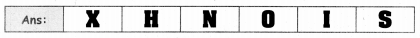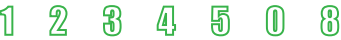Students can download 5th Maths Term 1 Chapter 1 Geometry Ex 1.2 Questions and Answers, Notes, Samacheer Kalvi 5th Maths Guide Pdf helps you to revise the complete Tamilnadu State Board New Syllabus, helps students complete homework assignments and to score high marks in board exams.

Tamilnadu Samacheer Kalvi 5th Maths Solutions Term 1 Chapter 1 Geometry Ex 1.2

Question 1.
Among the following shapes, find out which one would look the same after one-fourth turn. Put a (✓) mark.Question 2.
Among the following letters, find out which one would look same after half turn.Question 3.
Find the numbers which will look same on a half-turn